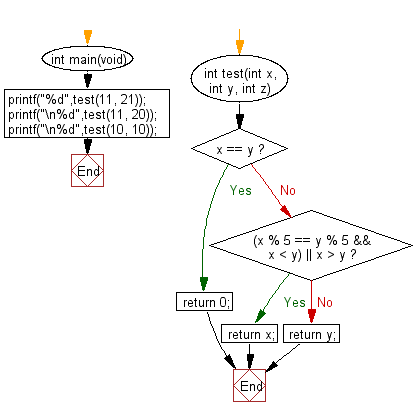﻿ C Program: Find the larger of two specified integers# C Exercises: Find the larger from two given integers of specified pattern

## C-programming basic algorithm: Exercise-27 with Solution

Write a C program to find the larger from two given integers. However if the two integers have the same remainder when divided by 5, then the return the smaller integer. If the two integers are the same, return 0.

C Code:

``````#include <stdio.h>
#include <stdlib.h>
int main(void){
printf("%d",test(11, 21));
printf("\n%d",test(11, 20));
printf("\n%d",test(10, 10));
}
int test(int x, int y, int z)
{
if (x == y)
{
return 0;
}
else if ((x % 5 == y % 5 && x < y) || x > y)
{
return x;
}
else
{
return y;
}
}
``````

Sample Output:

```11
20
0
```

Pictorial Presentation:Flowchart:C Programming Code Editor:

What is the difficulty level of this exercise?

Test your Programming skills with w3resource's quiz.

﻿

## C Programming: Tips of the Day

Why doesn't a+++++b work?

printf("%d",a+++++b); is interpreted as (a++)++ + b according to the Maximal Munch Rule!.

++ (postfix) doesn't evaluate to an lvalue but it requires its operand to be an lvalue.

! 6.4/4 says the next preprocessing token is the longest sequence of characters that could constitute a preprocessing token"

Ref : https://bit.ly/3fdldUT